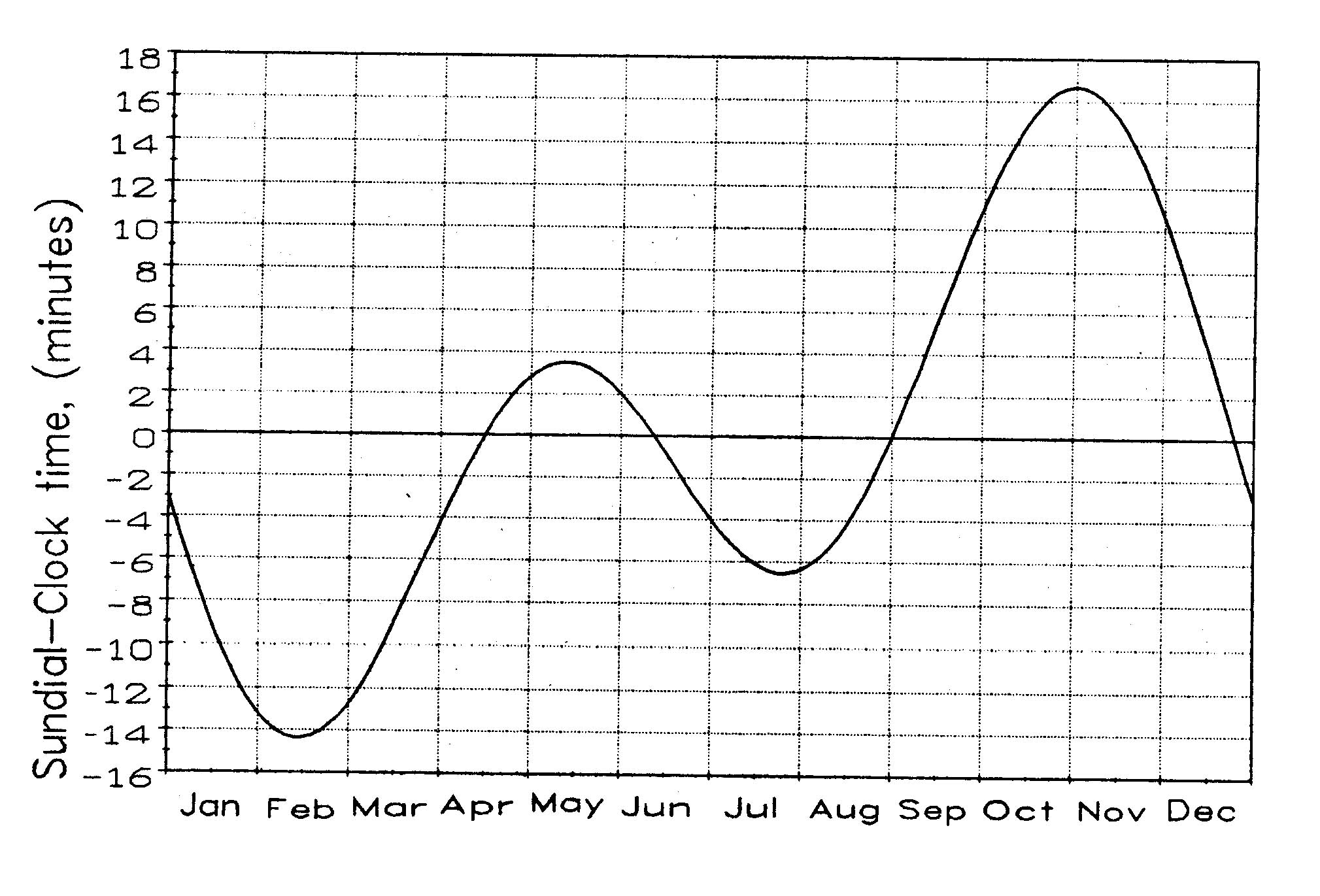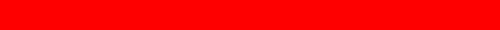# EQUATION OF TIME

Back ] Up ] Next ]I

n the two previous sections, we have seen how the difference between sundial time and standard time depends on two effects: the eccentricity of the Earth's orbit and the inclination of the Earth's orbit.

The combination of these two effects, which is the true equation of time, is plotted in Fig.6. In December and January these two effects are both working to slow the sundial time, while in June and July the two effects are opposed to each other. The sundial lags only six minutes during June when the two effects are opposed, but lags 13˝ minutes during December. The equation of time expresses the relationship between the sundial and standard time, and the standard time is then available from the sundial by applying the proper value, plus or minus, from the equation of time. But such conversion yields true standard time only if the sundial is on the standard meridian. One must know one's distance east or west of the standard meridian in order to make the remaining correction to the sundial time.

The Earth turns through one time zone in an hour. The time zone is 15 degrees wide (one twenty-fourth of 360 degrees), so each degree of longitude within the time zone is equivalent to four minutes of time (60 min./15o). This then is the correction to make for each degree of longitude away from the standard meridian: minus if east or plus if west of the standard meridian

As an example, suppose that you are located at longitude 155 degrees west. What is the correction to arrive at standard time for your time zone? The standard meridian is the 150 degree west meridian, so you are located 5 degrees west of that. Every degree is 4 minutes of time, so the sun passes overhead at your longitude 4 x 5 = 20 minutes later than at the standard meridian. Thus, you must add 20 minutes from your sundial time to get the standard zone time. This, of course, is in addition to the time that must be added or subtracted according to the equation of time. See Appendix B for additional examples.Figure 6

The Equation of Time

 [ Back ] [ Up ] [ Next ]